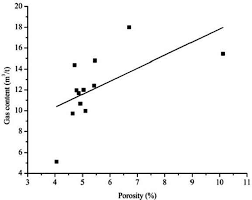## How to Calculate and Solve for Gas Pore Volume of a Reservoir Fluid Flow | The Calculator EncyclopediaThe image above represents the gas pore volume.

To compute for the gas pore volume, three essential parameters are needed and these parameters are Initial Gas FVF (Bgi), Initial Water Saturation (Swi) and Initial Gas in Place (G).

The formula for calculating the gas pore volume:

(PV)g = GBgi / 1 – Swi

Where;

(PV)g = Gas Pore Volume
Bgi = Initial Gas FVF
Swi = Initial Water Saturation
G = Initial Gas in Place

Let’s solve an example;
Find the gas pore volume when the initial gas FVF is 12, initial water saturation is 9 and initial gas in place is 13.

This implies that;

Bgi = Initial Gas FVF = 12
Swi = Initial Water Saturation = 9
G = Initial Gas in Place = 13

(PV)g = GBgi / 1 – Swi
(PV)g = 13 x 12 / 1 – 9
(PV)g = 156 / -8
(PV)g =  -19.5

Therefore, the gas pore volume is -19.5

Calculating the Initial Gas FVF when the Gas Pore Volume, Initial Water Saturation and Initial Gas in Place is Given.

Bgi = (PV)g (1 – Swi) / G

Where;

Bgi = Initial Gas FVF
(PV)g = Gas Pore Volume
Swi = Initial Water Saturation
G = Initial Gas in Place

Let’s solve an example;
Find the initial gas FVF when the gas pore volume is 19, initial water saturation is 11 and initial gas in place is 16.

This implies that;

(PV)g = Gas Pore Volume = 19
Swi = Initial Water Saturation = 11
G = Initial Gas in Place = 16

Bgi = (PV)g (1 – Swi) / G
Bgi = 19 (1 – 11) / 16
Bgi = 19 (-10) / 16
Bgi = -190 / 16
Bgi = -11.875

Therefore, the initial gas FVF is -11.875.

Calculating the Initial Water Saturation when the Gas Pore Volume, Initial Gas FVF and Initial Gas in Place is Given.

Swi = 1 – GBgi / (PV)g

Where;

Swi = Initial Water Saturation
G = Initial Gas in Place
(PV)g = Gas Pore Volume
Bgi = Initial Gas FVF

Let’s solve an example;
Find the initial water saturation when the initial gas FVF is 24, initial gas in place is 6 and gas pore volume is 46.

This implies that;

G = Initial Gas in Place = 6
(PV)g = Gas Pore Volume = 46
Bgi = Initial Gas FVF = 24

Swi = 1 – GBgi / (PV)g
Swi = 1 – 6 x 24 / 46
Swi = 1 – 144 / 46
Swi = 1 – 3.13
Swi = -2.13

Therefore, the initial water saturation is -2.13.